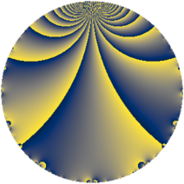# Properties

 Label 1143.3.bLevel $1143$ Weight $3$ Character orbit 1143.b Rep. character $\chi_{1143}(890,\cdot)$ Character field $\Q$ Dimension $84$ Newform subspaces $1$ Sturm bound $384$ Trace bound $0$

# Related objects

## Defining parameters

 Level: $$N$$ $$=$$ $$1143 = 3^{2} \cdot 127$$ Weight: $$k$$ $$=$$ $$3$$ Character orbit: $$[\chi]$$ $$=$$ 1143.b (of order $$2$$ and degree $$1$$) Character conductor: $$\operatorname{cond}(\chi)$$ $$=$$ $$3$$ Character field: $$\Q$$ Newform subspaces: $$1$$ Sturm bound: $$384$$ Trace bound: $$0$$

## Dimensions

The following table gives the dimensions of various subspaces of $$M_{3}(1143, [\chi])$$.

Total New Old
Modular forms 260 84 176
Cusp forms 252 84 168
Eisenstein series 8 0 8

## Trace form

 $$84q - 160q^{4} + O(q^{10})$$ $$84q - 160q^{4} - 48q^{10} + 16q^{13} + 360q^{16} + 64q^{19} - 8q^{22} - 388q^{25} - 120q^{28} - 160q^{31} + 192q^{34} - 152q^{37} + 208q^{40} - 24q^{43} + 56q^{46} + 564q^{49} - 80q^{52} + 136q^{55} - 136q^{58} + 168q^{61} - 736q^{64} + 168q^{67} - 608q^{70} + 80q^{73} - 32q^{76} - 168q^{79} + 528q^{82} + 288q^{85} - 392q^{88} + 176q^{91} + 176q^{94} - 120q^{97} + O(q^{100})$$

## Decomposition of $$S_{3}^{\mathrm{new}}(1143, [\chi])$$ into newform subspaces

Label Dim. $$A$$ Field CM Traces $q$-expansion
$$a_2$$ $$a_3$$ $$a_5$$ $$a_7$$
1143.3.b.a $$84$$ $$31.144$$ None $$0$$ $$0$$ $$0$$ $$0$$

## Decomposition of $$S_{3}^{\mathrm{old}}(1143, [\chi])$$ into lower level spaces

$$S_{3}^{\mathrm{old}}(1143, [\chi]) \cong$$ $$S_{3}^{\mathrm{new}}(381, [\chi])$$$$^{\oplus 2}$$